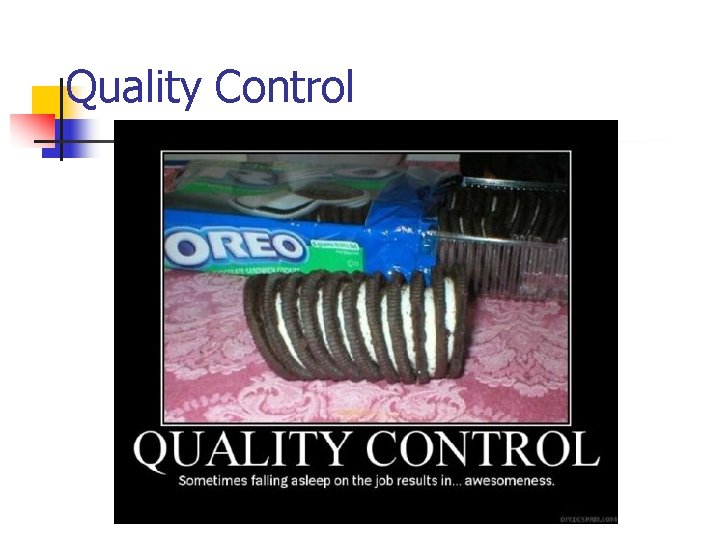# Statistical Quality Control by R B Clough UNH

• Slides: 51Statistical Quality Control by R. B. Clough – UNH M. E. Henrie - UAA Statistical Quality ControlThe Right Statistical Tools Statistical Quality Control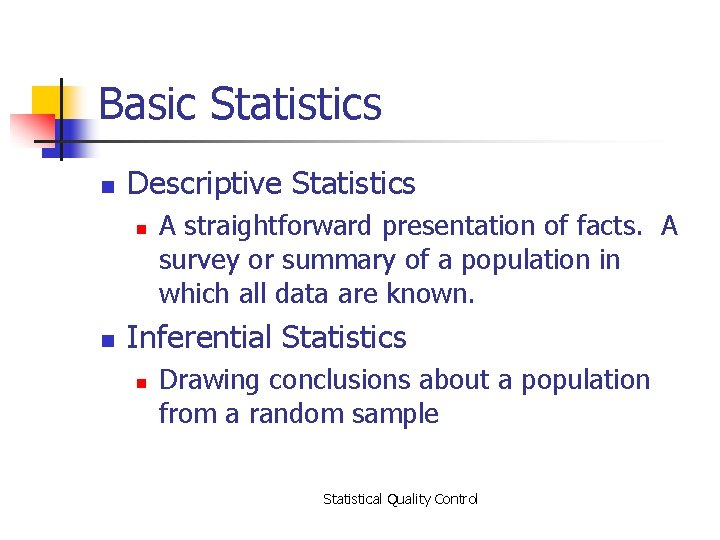Basic Statistics n Descriptive Statistics n n A straightforward presentation of facts. A survey or summary of a population in which all data are known. Inferential Statistics n Drawing conclusions about a population from a random sample Statistical Quality Control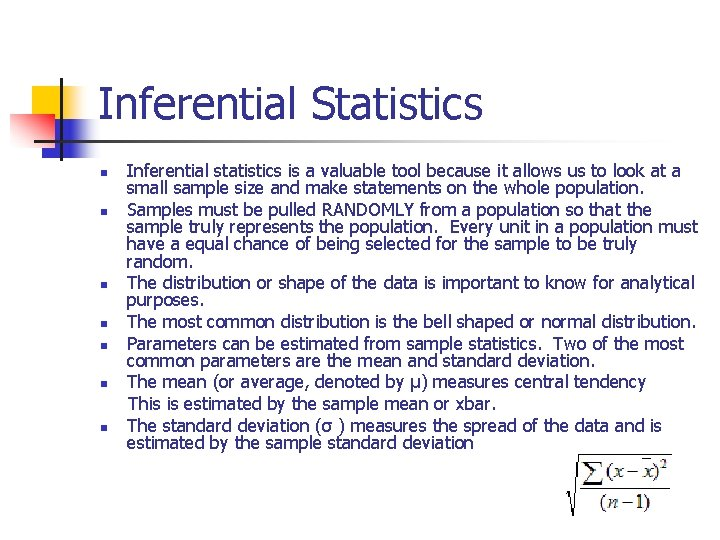Inferential Statistics n n n n Inferential statistics is a valuable tool because it allows us to look at a small sample size and make statements on the whole population. Samples must be pulled RANDOMLY from a population so that the sample truly represents the population. Every unit in a population must have a equal chance of being selected for the sample to be truly random. The distribution or shape of the data is important to know for analytical purposes. The most common distribution is the bell shaped or normal distribution. Parameters can be estimated from sample statistics. Two of the most common parameters are the mean and standard deviation. The mean (or average, denoted by μ) measures central tendency This is estimated by the sample mean or xbar. The standard deviation (σ ) measures the spread of the data and is estimated by the sample standard deviation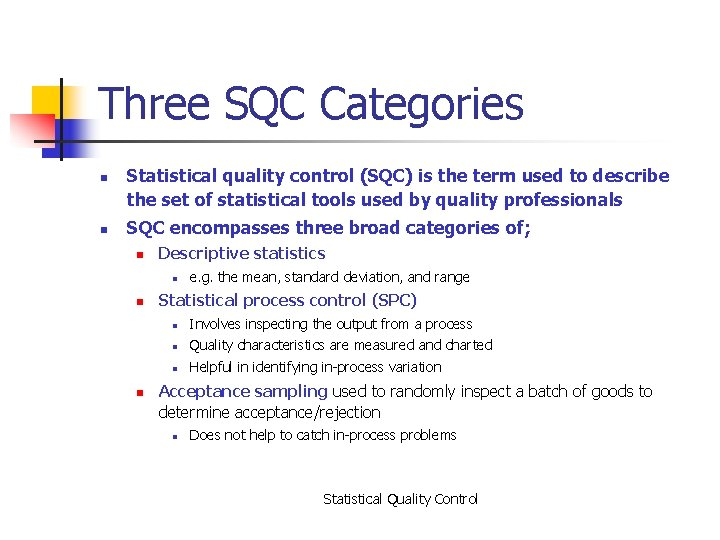Three SQC Categories n n Statistical quality control (SQC) is the term used to describe the set of statistical tools used by quality professionals SQC encompasses three broad categories of; n Descriptive statistics n n n e. g. the mean, standard deviation, and range Statistical process control (SPC) n Involves inspecting the output from a process n Quality characteristics are measured and charted n Helpful in identifying in-process variation Acceptance sampling used to randomly inspect a batch of goods to determine acceptance/rejection n Does not help to catch in-process problems Statistical Quality Control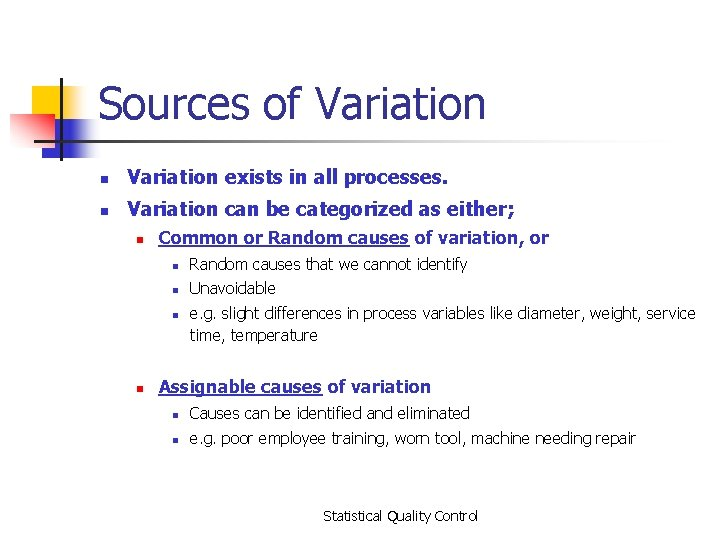Sources of Variation n Variation exists in all processes. n Variation can be categorized as either; n Common or Random causes of variation, or n Random causes that we cannot identify n Unavoidable n n e. g. slight differences in process variables like diameter, weight, service time, temperature Assignable causes of variation n Causes can be identified and eliminated n e. g. poor employee training, worn tool, machine needing repair Statistical Quality Control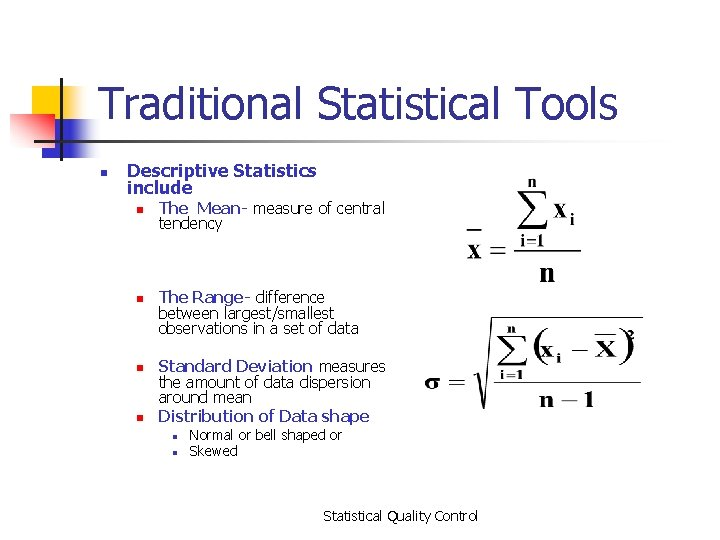Traditional Statistical Tools n Descriptive Statistics include n n The Mean- measure of central tendency The Range- difference between largest/smallest observations in a set of data Standard Deviation measures the amount of data dispersion around mean Distribution of Data shape n n Normal or bell shaped or Skewed Statistical Quality ControlDistribution of Data n Normal distributions n Skewed distribution Statistical Quality Control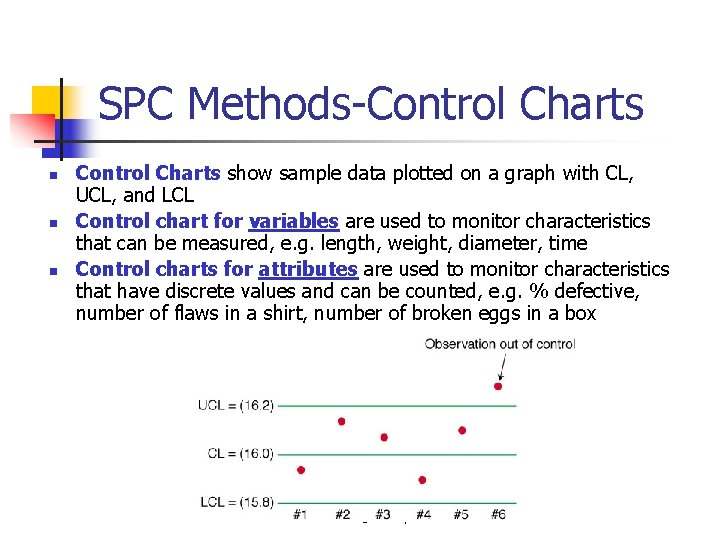SPC Methods-Control Charts n n n Control Charts show sample data plotted on a graph with CL, UCL, and LCL Control chart for variables are used to monitor characteristics that can be measured, e. g. length, weight, diameter, time Control charts for attributes are used to monitor characteristics that have discrete values and can be counted, e. g. % defective, number of flaws in a shirt, number of broken eggs in a box © Wiley 2010Analysis of Patterns on Control Charts n n n When do you have a problem with your process? One or more points outside of the control limits A run of at least seven points (up, down or above or below center line) Two or three consecutive points outside the 2 -sigma warning limits, but still inside the control limits Four or five consecutive points beyond the 1 -sigma limits An unusual or nonrandom pattern in the data From Douglas C. Montgomery “Introduction to Statistical Quality Control”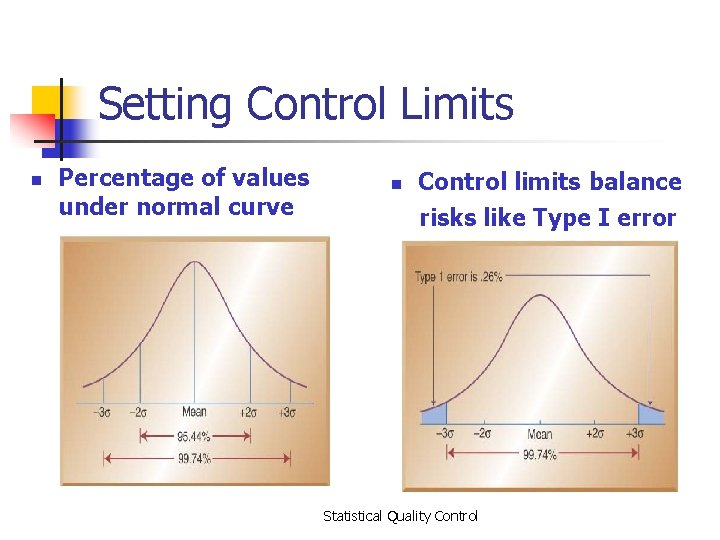Setting Control Limits n Percentage of values under normal curve n Control limits balance risks like Type I error Statistical Quality Control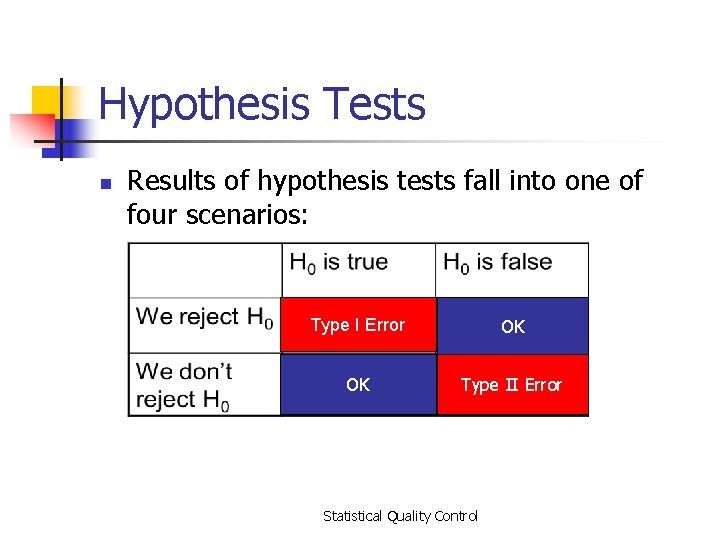Hypothesis Tests n Results of hypothesis tests fall into one of four scenarios: Type I Error OK OK Type II Error Statistical Quality Control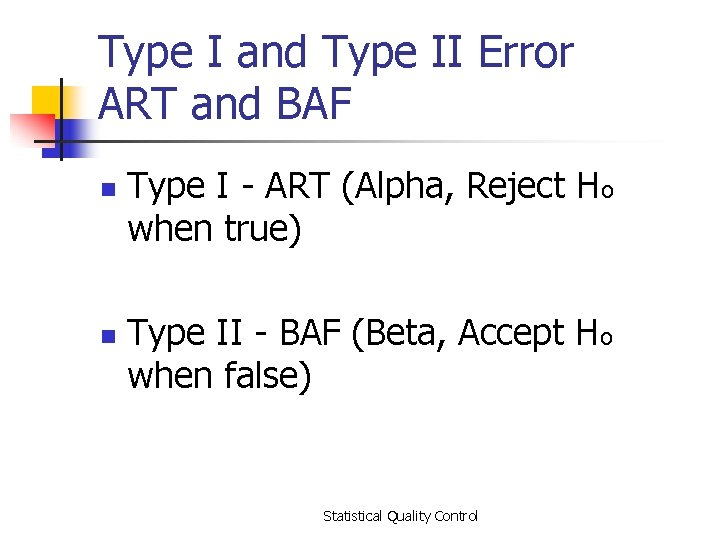Type I and Type II Error ART and BAF n n Type I - ART (Alpha, Reject Ho when true) Type II - BAF (Beta, Accept Ho when false) Statistical Quality Control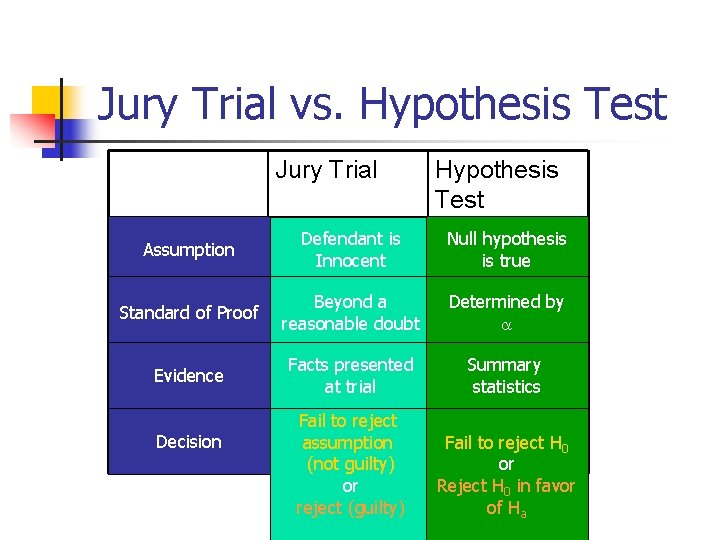Jury Trial vs. Hypothesis Test Jury Trial Hypothesis Test Assumption Defendant is Innocent Null hypothesis is true Standard of Proof Beyond a reasonable doubt Determined by Evidence Facts presented at trial Summary statistics Decision Fail to reject assumption Fail to reject H 0 (not guilty) or or Reject H 0 in favor reject (guilty) of Ha © Wiley 2007Context? n n n What does it mean to make a type I error here? n Convict an innocent person of a crime. What does it mean to make a type II error? n Fail to convict a guilty person. What do we usually say about type I and type II error rates in this context?Control Charts for Variables n n Use x-bar and R-bar charts together Used to monitor different variables X-bar & R-bar Charts reveal different problems In statistical control on one chart, out of control on the other chart? OK? Statistical Quality ControlControl Charts for Variables n n Use x-bar charts to monitor the changes in the mean of a process (central tendencies) Use R-bar charts to monitor the dispersion or variability of the process System can show acceptable central tendencies but unacceptable variability or System can show acceptable variability but unacceptable central tendencies Statistical Quality ControlGraphical Analysis “A picture is worth a thousand words. ” n Graphical analysis is the first step in analyzing your data. Examples: n n n Distribution (histogram, dotplot, boxplot) Time Series plot for trending I-chart (for Individual data points) Normality Cpk (when applicable) graph (Minitab) Statistical Quality ControlDotplot of Tensile Test Data Statistical Quality Control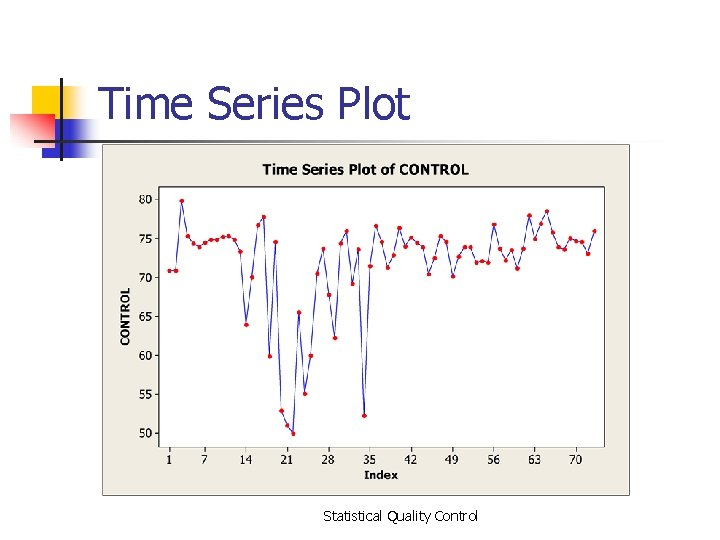Time Series Plot Statistical Quality ControlIndividuals (I) Chart Statistical Quality ControlNormal Probability Plot Statistical Quality ControlCpk Graph (Minitab) Statistical Quality ControlConfidence Statements n n n A confidence statement is used to state the level of quality of manufactured product. Whether it is dimensional or pass/fail data, confidence statements can help to state the quality level achieved by a process in relation to the specification. When the true means and standard deviations are not known, estimates of these parameters such as sample standard deviations and sample means are used to make confidence statements based on tolerance limits using either binomial probabilities or k -factors. There are three types of confidence statements that are primarily used. Statistical Quality Control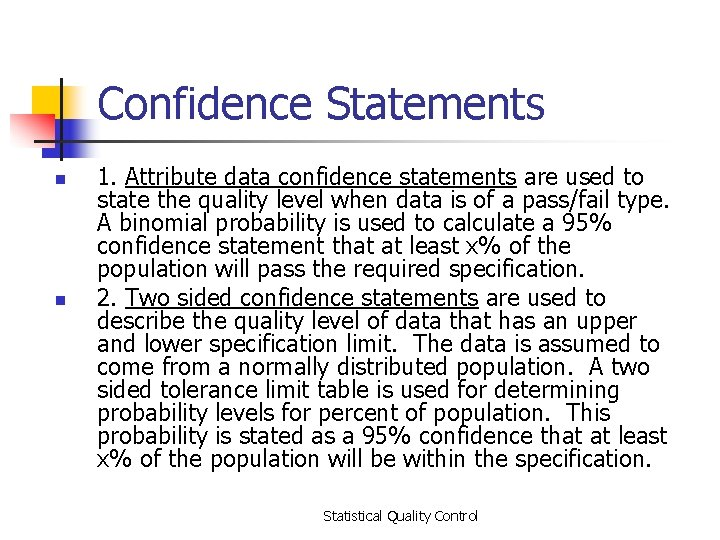Confidence Statements n n 1. Attribute data confidence statements are used to state the quality level when data is of a pass/fail type. A binomial probability is used to calculate a 95% confidence statement that at least x% of the population will pass the required specification. 2. Two sided confidence statements are used to describe the quality level of data that has an upper and lower specification limit. The data is assumed to come from a normally distributed population. A two sided tolerance limit table is used for determining probability levels for percent of population. This probability is stated as a 95% confidence that at least x% of the population will be within the specification. Statistical Quality Control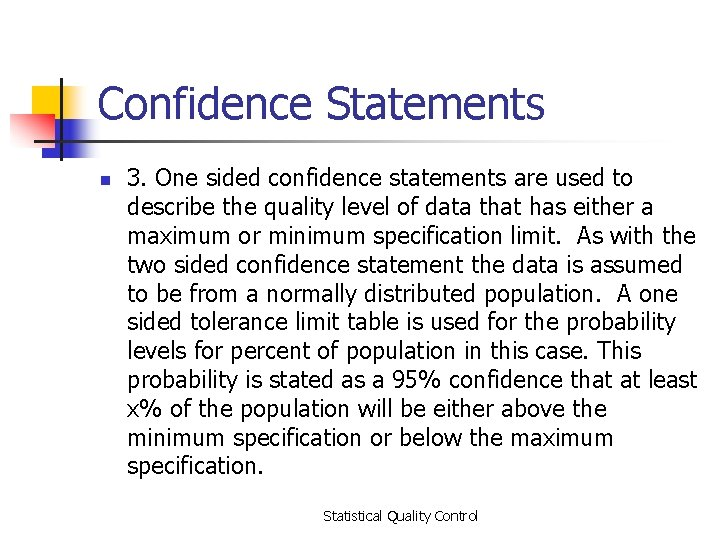Confidence Statements n 3. One sided confidence statements are used to describe the quality level of data that has either a maximum or minimum specification limit. As with the two sided confidence statement the data is assumed to be from a normally distributed population. A one sided tolerance limit table is used for the probability levels for percent of population in this case. This probability is stated as a 95% confidence that at least x% of the population will be either above the minimum specification or below the maximum specification. Statistical Quality ControlConfidence Statements For confidence that data is greater than min spec Sample mean – K*(sample sd) = min spec xbar- Ks = min - xbar K = (xbar - min)/s For confidence that data is less than max spec Sample mean + K*(sample sd) = max spec xbar + Ks = max - xbar K = (max - xbar)/s For two sided tolerance limit both calculations should be made and lowest k-factor compared with table value. Statistical Quality ControlConfidence Statements Statistical Quality Control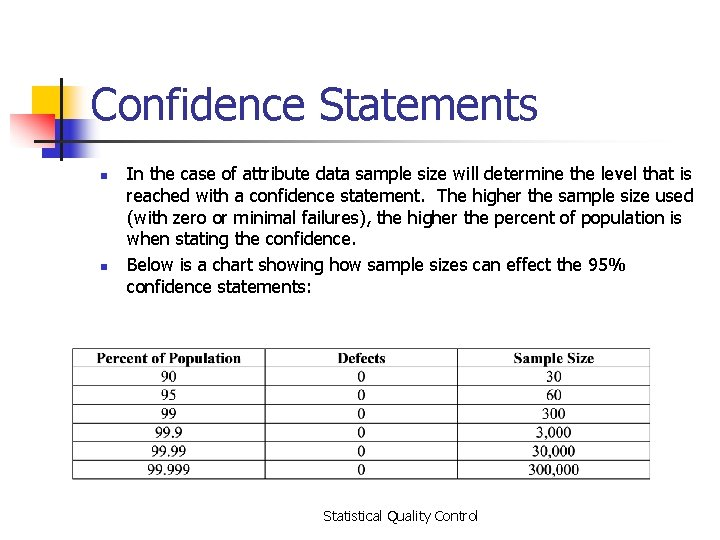Confidence Statements n n In the case of attribute data sample size will determine the level that is reached with a confidence statement. The higher the sample size used (with zero or minimal failures), the higher the percent of population is when stating the confidence. Below is a chart showing how sample sizes can effect the 95% confidence statements: Statistical Quality ControlSample Size n The following simple formula may be used to estimate sample size (for any distribution) to determine a sample mean, or average, when estimates of the standard deviation are known. Statistical Quality ControlSample Size The following simple formula may be used to estimate sample size to determine a proportion (fraction) defective. n= p (1 -p) (z / B) Where: n Statistical Quality Control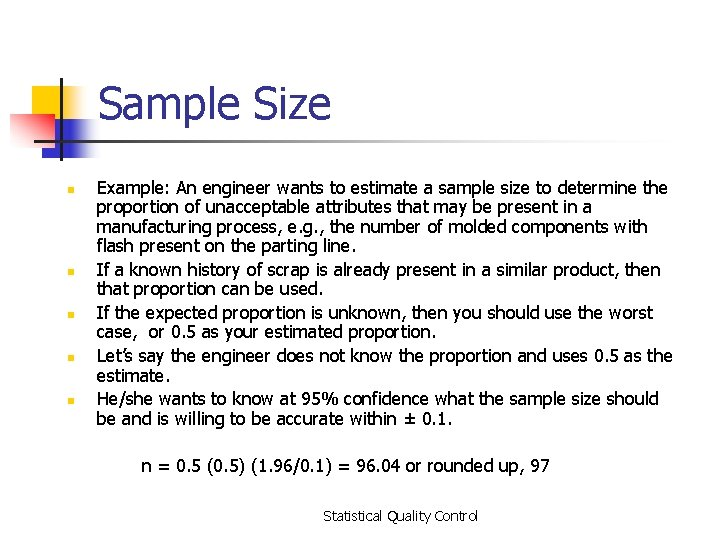Sample Size n n n Example: An engineer wants to estimate a sample size to determine the proportion of unacceptable attributes that may be present in a manufacturing process, e. g. , the number of molded components with flash present on the parting line. If a known history of scrap is already present in a similar product, then that proportion can be used. If the expected proportion is unknown, then you should use the worst case, or 0. 5 as your estimated proportion. Let’s say the engineer does not know the proportion and uses 0. 5 as the estimate. He/she wants to know at 95% confidence what the sample size should be and is willing to be accurate within ± 0. 1. n = 0. 5 (0. 5) (1. 96/0. 1) = 96. 04 or rounded up, 97 Statistical Quality Control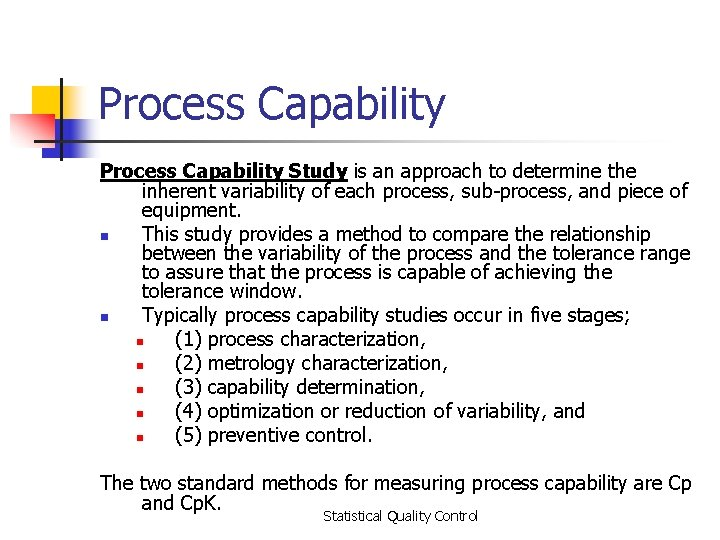Process Capability Study is an approach to determine the inherent variability of each process, sub-process, and piece of equipment. n This study provides a method to compare the relationship between the variability of the process and the tolerance range to assure that the process is capable of achieving the tolerance window. n Typically process capability studies occur in five stages; n (1) process characterization, n (2) metrology characterization, n (3) capability determination, n (4) optimization or reduction of variability, and n (5) preventive control. The two standard methods for measuring process capability are Cp and Cp. K. Statistical Quality Control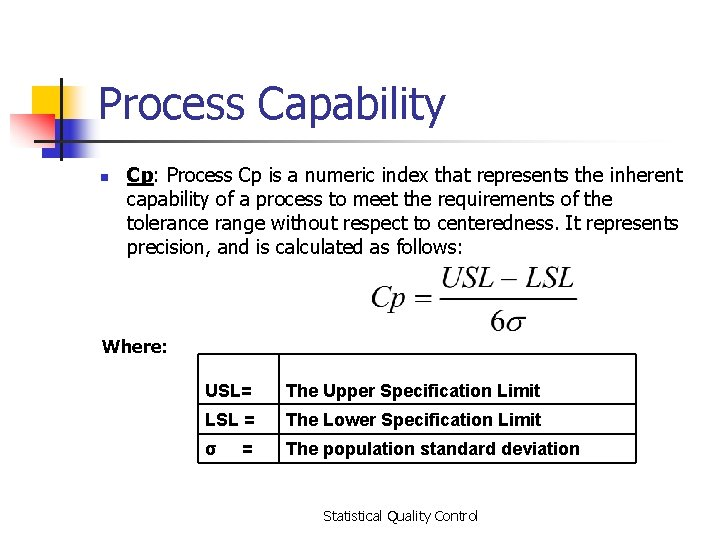Process Capability n Cp: Process Cp is a numeric index that represents the inherent capability of a process to meet the requirements of the tolerance range without respect to centeredness. It represents precision, and is calculated as follows: Where: USL= The Upper Specification Limit LSL = The Lower Specification Limit σ The population standard deviation = Statistical Quality ControlProcess Capability n Cp represents the precision, but not the accuracy of the process in respect to the tolerance window. High Accuracy but low precision High Precision but low Accuracy Statistical Quality Control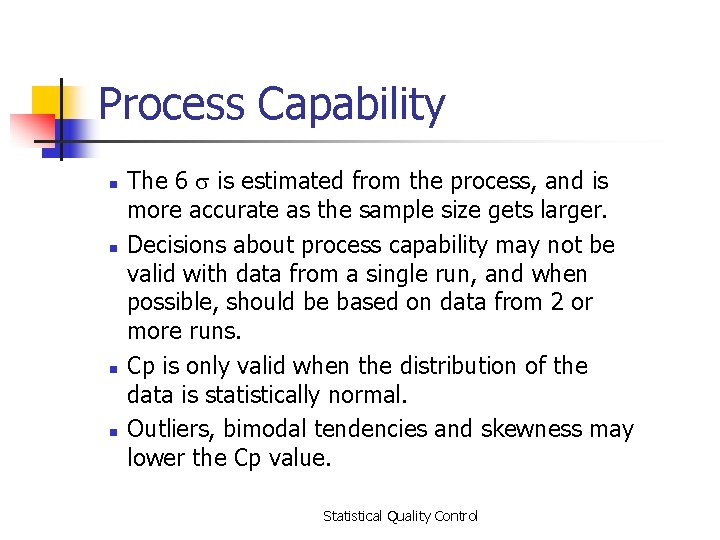Process Capability n n The 6 is estimated from the process, and is more accurate as the sample size gets larger. Decisions about process capability may not be valid with data from a single run, and when possible, should be based on data from 2 or more runs. Cp is only valid when the distribution of the data is statistically normal. Outliers, bimodal tendencies and skewness may lower the Cp value. Statistical Quality Control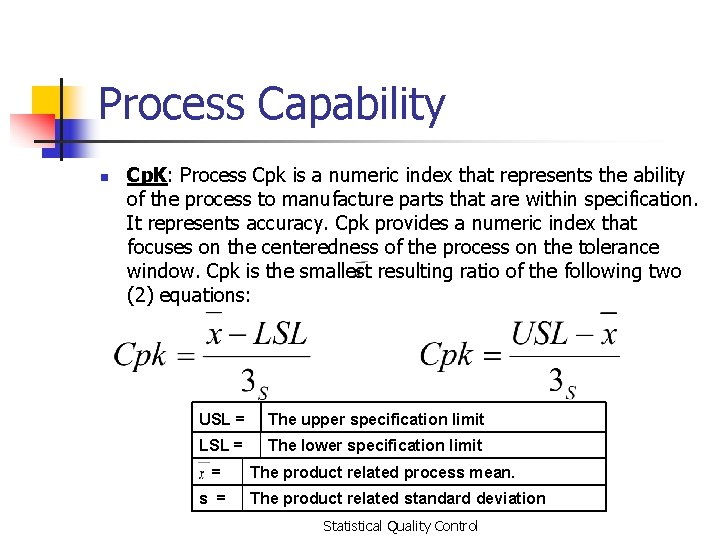Process Capability n Cp. K: Process Cpk is a numeric index that represents the ability of the process to manufacture parts that are within specification. It represents accuracy. Cpk provides a numeric index that focuses on the centeredness of the process on the tolerance window. Cpk is the smallest resulting ratio of the following two (2) equations: USL = The upper specification limit LSL = The lower specification limit = s = The product related process mean. The product related standard deviation Statistical Quality Control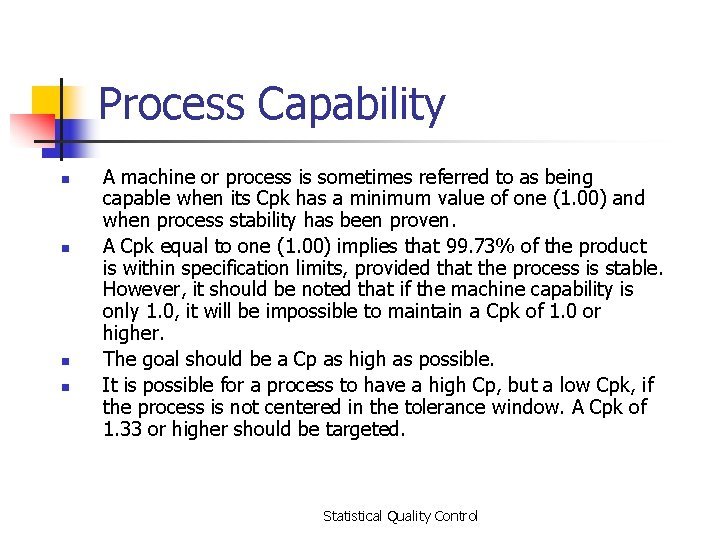Process Capability n n A machine or process is sometimes referred to as being capable when its Cpk has a minimum value of one (1. 00) and when process stability has been proven. A Cpk equal to one (1. 00) implies that 99. 73% of the product is within specification limits, provided that the process is stable. However, it should be noted that if the machine capability is only 1. 0, it will be impossible to maintain a Cpk of 1. 0 or higher. The goal should be a Cp as high as possible. It is possible for a process to have a high Cp, but a low Cpk, if the process is not centered in the tolerance window. A Cpk of 1. 33 or higher should be targeted. Statistical Quality ControlCp. K n n A Cp. K of 1. 33 means that the difference between the mean and specification limit is 4σ (since 1. 33 is 4/3). With a Cp. K of 1. 33, 99. 994% of the product is within the within specification. Similarly a Cp. K of 2. 0 is 6σ between the mean and specification limit (since 2. 0 is 6/3). With a Cp. K of 2. 0 99. 9999998% of the product is within specification. Statistical Quality ControlAcceptance Sampling n n Definition: the third branch of SQC refers to the process of randomly inspecting a certain number of items from a lot or batch in order to decide whether to accept or reject the entire batch Different from SPC because acceptance sampling is performed either before or after the process rather than during n n n Sampling before typically is done to supplier material Sampling after involves sampling finished items before shipment or finished components prior to assembly Used where inspection is expensive, volume is high, or inspection is destructive Statistical Quality ControlAcceptance Sampling Plans n n Goal of Acceptance Sampling plans is to determine the criteria for acceptance or rejection based on: n Size of the lot (N) n Size of the sample (n) n Number of defects above which a lot will be rejected (c) n Level of confidence we wish to attain There are single, double, and multiple sampling plans n n Which one to use is based on cost involved, time consumed, and cost of passing on a defective item Can be used on either variable or attribute measures, but more commonly used for attributes Statistical Quality ControlAcceptance Sampling Plans n n ANSI/ASQC Z 1. 4 (Attribute or P/F Data) ANSI/ASQC Z 1. 9 (Variable Data) C=0 (Attribute, reject on 1) MIL STD 1235 C (Continuous Production) Statistical Quality Control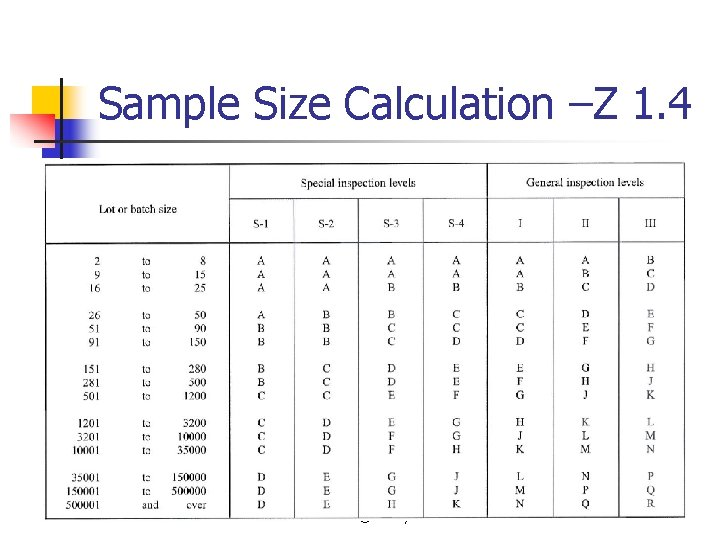Sample Size Calculation –Z 1. 4 © Wiley 2007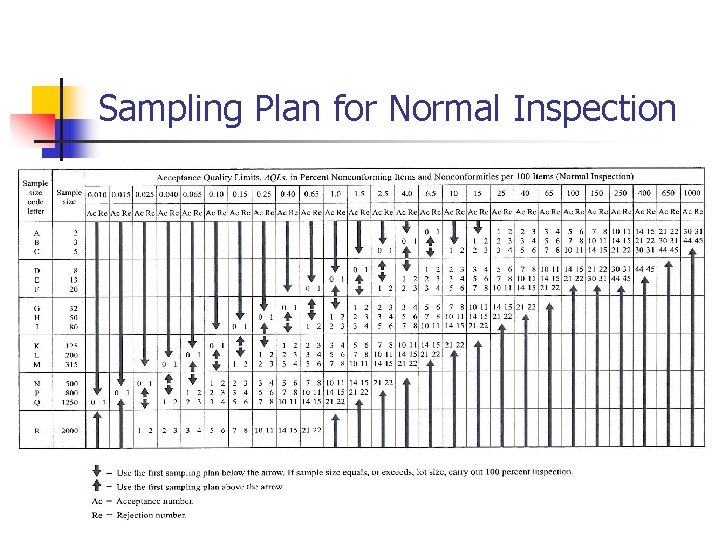Sampling Plan for Normal Inspection © Wiley 2007AQL Inspector’s Rule © Wiley 2007AQL Inspector’s Rule Accept/Reject Sample Size © Wiley 2007Acceptance Sampling Plans As mentioned acceptance sampling can reject “good” lots and accept “bad” lots. More formally: Producers risk refers to the probability of rejecting a good lot. In order to calculate this probability there must be a numerical definition as to what constitutes “good” • – • AQL (Acceptable Quality Limit) - the numerical definition of a good lot. The ANSI/ASQC standard describes AQL as “the maximum percentage or proportion of nonconforming items or number of nonconformities in a batch that can be considered satisfactory as a process average” Consumers Risk refers to the probability of accepting a bad lot where: – LTPD (Lot Tolerance Percent Defective) - the numerical definition of a bad lot described by the ANSI/ASQC standard as “the percentage or proportion of nonconforming items or noncomformities in a batch for which the customer wishes the probability of acceptance to be a specified low value. Statistical Quality Control